SLOS358C September   2011  – April 2020

PRODUCTION DATA.

1. Features
2. Applications
3. Description
4. Revision History
5. Packaging and Ordering Information
6. Electrical Specifications
7. Device Information
1. 7.1 PIN Configurations
8. Table of Graphs
9. Typical Characteristics: VS = 2.7 V
10. 10Typical Characteristics: VS = 5 V
11. 11Application Information
12. 12Device and Documentation Support
13. 13Mechanical, Packaging, and Orderable Information

• RUN|10
• D|8
• DGK|8
• DGK|8

## 9 Typical Characteristics: VS = 2.7 V

Test conditions unless otherwise noted: VS+ = 2.7 V, VS– = 0 -, CM = open, VOUT = 2 Vpp, RF = 2 kΩ, RL = 2 kΩ differential, G = 1 V/V, single-ended input, differential output, input and output referenced to mid-supply unless otherwise noted.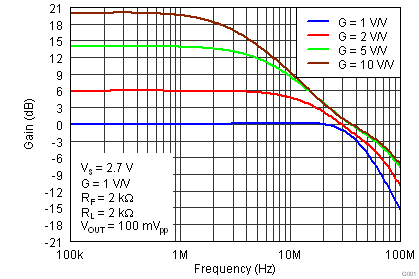Figure 1. Small-Signal Frequency Response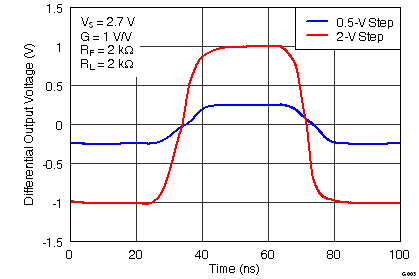Figure 3. Large and Small-Signal Pulse Response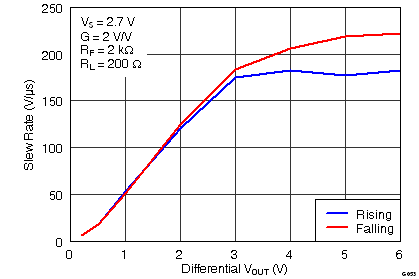Figure 5. Differential Slew Rate vs VOUT StepFigure 7. 10 kHz FFT On Audio AnalyzerFigure 9. Harmonic Distortion vs Output Voltage at 1 MHz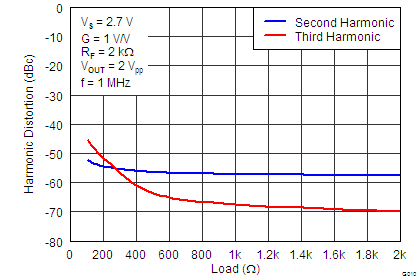Figure 11. Harmonic Distortion vs Load at 1 MHzFigure 13. Two-Tone, 2nd and 3rd Order Intermodulation Distortion vs FrequencyFigure 15. Single-Ended Output Saturation Voltage vs Load Current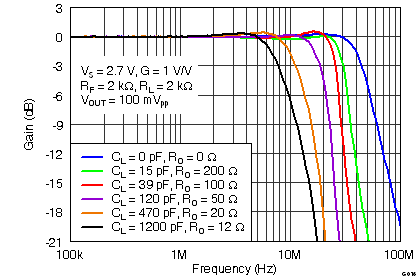Figure 17. Frequency Response vs CLOAD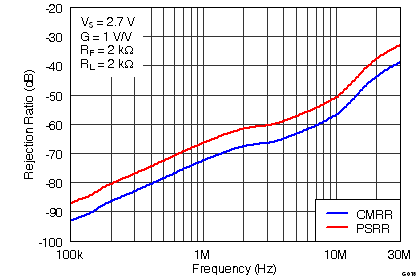Figure 19. Rejection Ratio vs Frequency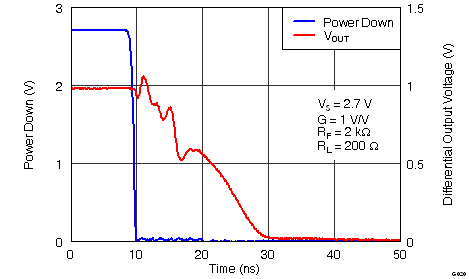Figure 21. Turn-Off Time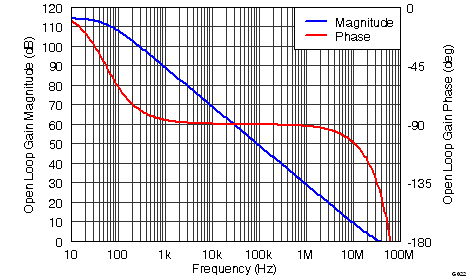Figure 23. Main Amplifier Differential Open-Loop Gain and Phase vs Frequency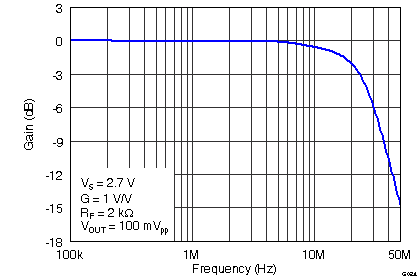Figure 25. VOCM Small-Signal Frequency Response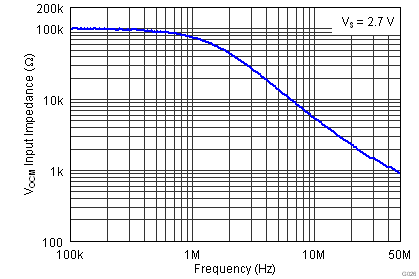Figure 27. VOCM Input Impedance vs Frequency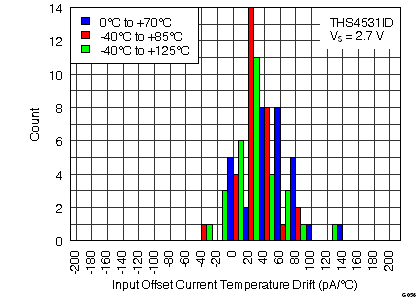Figure 29. Input Offset Current Temperature Drift Histogram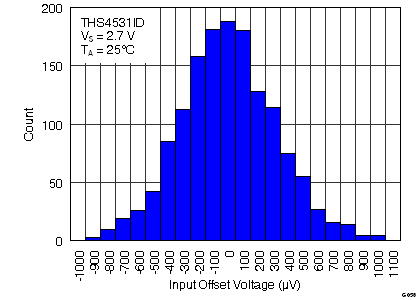Figure 31. Input Offset Voltage Histogram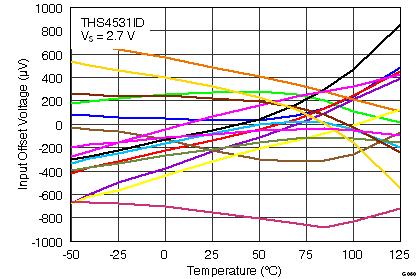Figure 33. Input Offset Voltage vs Temperature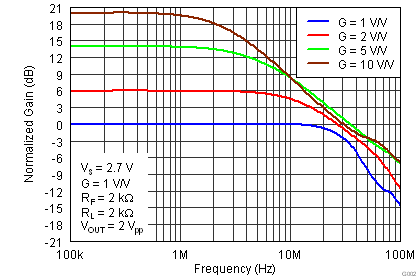Figure 2. Large-Signal Frequency Response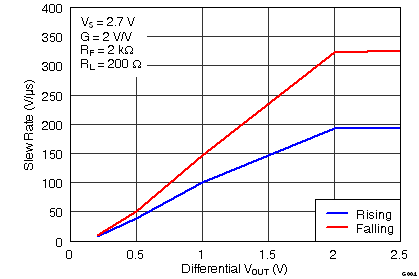Figure 4. Single-Snded Slew Rate vs VOUT Step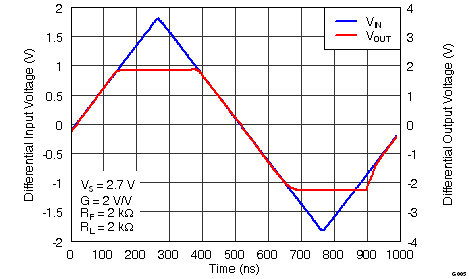Figure 6. Overdrive Recovery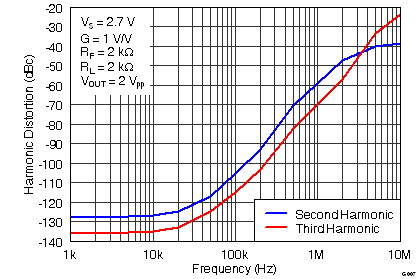Figure 8. Harmonic Distortion vs Frequency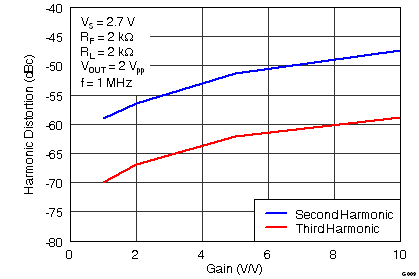Figure 10. Harmonic Distortion vs GAIN at 1 MHz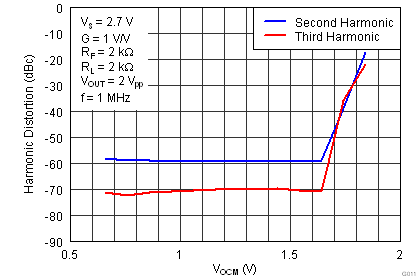Figure 12. Harmonic Distortion vs VOCM at 1 MHzFigure 14. Single-Ended Output Voltage Swing vs Load Resistance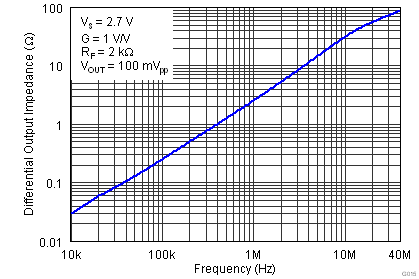Figure 16. Main Amplifier Differential Output Impedance vs FrequencyFigure 18. RO vs CLOAD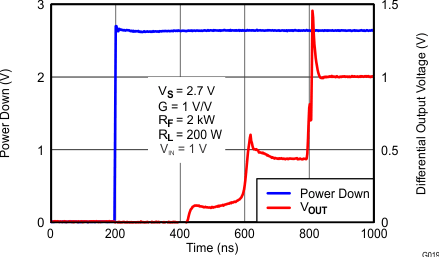Figure 20. Turn-On TimeFigure 22. Input-referred Voltage Noise and Current Noise Spectral Density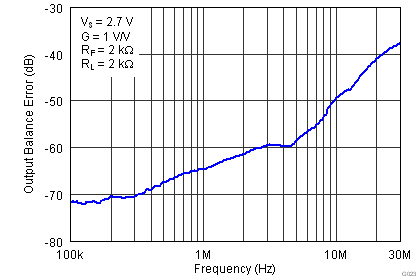Figure 24. Output Balance Error vs Frequency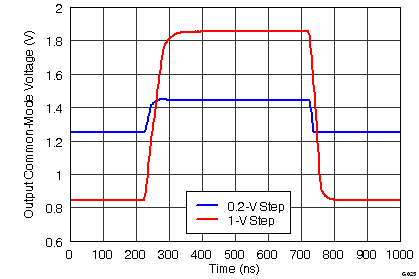Figure 26. VOCM Large and Small-Signal Pulse Response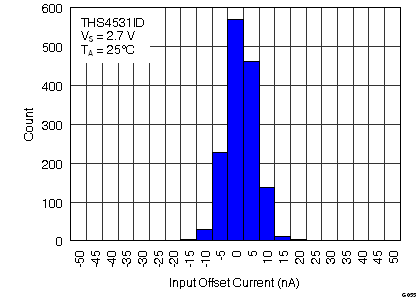Figure 28. Input Offset Current HistogramFigure 30. Input Offset Current vs Temperature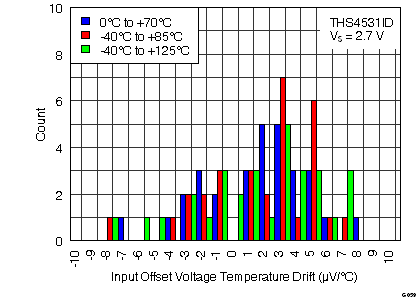Figure 32. Input Offset Voltage Temperature Drift Histogram